# The Poetry of Mathematics

Published:

Mathematicians often talk about the "beauty of mathematics". This project explores this statement through erasure poetry, with current mathematical texts* as the source material. I enjoy the process of writing poetry and the nature of an erasure poem, like a tricky word puzzle, appeals to me.

My aim is to create one poem every fortnight and release it every other Wednesday. Hopefully you will enjoy the (amateur!) verse maybe learn a thing or two and, with luck, my poetry will improve over the weeks! If you feel inspired, have any comments or would just like to have a go yourself, then please do feel free to contact me via email with your query or submission. Enjoy!

* Use of copyrighted material has been deemed "fair dealing" as outlined by UK Government's Exceptions to Copyright

Poem 001: The Matrix (5th July 2023)
• The Mathematical Topic
• Linear algebra: this branch of mathematics developed from trying to solve numerous linear equations all at once! The new objects used in this pursuit and examining the underlying structures at play led to this powerful and highly applicable field of mathematics. It is where most students first encounter the concept of a matrix, an object made of numbers but which does not behave like "normal" numbers. For example, the order in which we multiply two matrices together matters, as you may get different results depending on which way round you do it! (Compare this with multiplying two "normal" numbers where the order doesn't matter: $2\times 3$ is the same as $3\times 2$).

• The Book
• Elementary Linear Algebra (7th edition)
Howard Anton (1994)
John Wiley & Sons: New York, NY

(The poem uses page 25 from the seventh edition)

• The Poem: "The Matrix"
•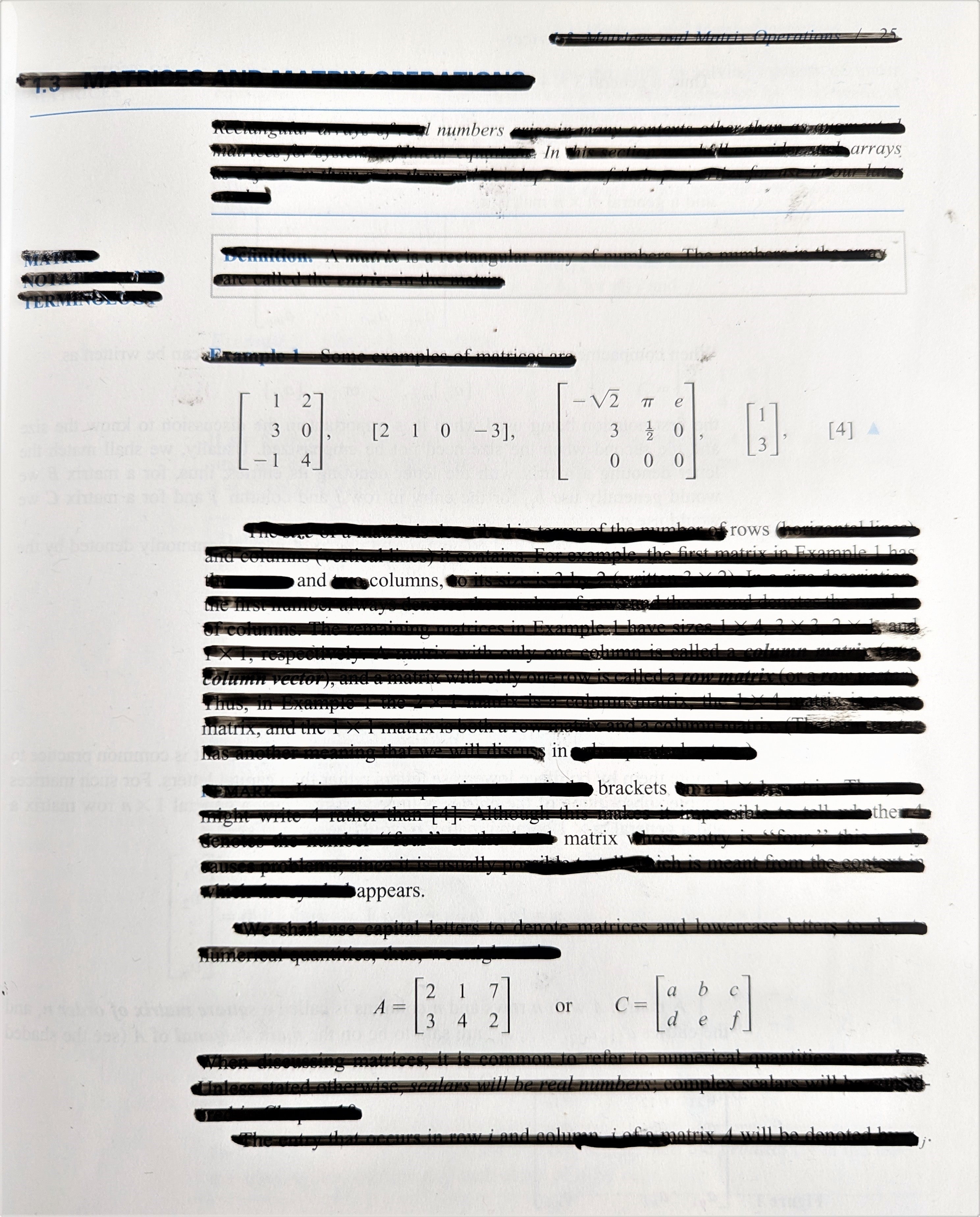"The Matrix"
Numbers in arrays.
Rows and columns in brackets.
The matrix appears.
The poem above is a haiku, a Japanese short-form poem that is a personal favourite. Due to its short structure, they are a very handy style when creating erasure poems, so I am sure many more haikus will appear (have a go at one yourself!).

Note: no books were harmed in the making of this poem, thanks to the helpful use of an acetate sheet.

Poem 002: A Real Challenge (19th July 2023)
• The Mathematical Topic
• Real analysis: a fundamental branch of mathematics where students take a peek behind the curtain to understand the theory behind calculus. The topic is introduced in the first year of the majority of mathematics degrees and contains the first "new" material encountered by undergraduate students (real analysis is not part of the standard A-level syllabus). Consequently, it is often considered a "difficult" subject and provides a challenge for most students.

• The Book
• Understanding Analysis
Stephen Abbott (2010)
Springer: New York, NY

(The poem uses page 76 from the first edition)

• The Poem: "A Real Challenge"
•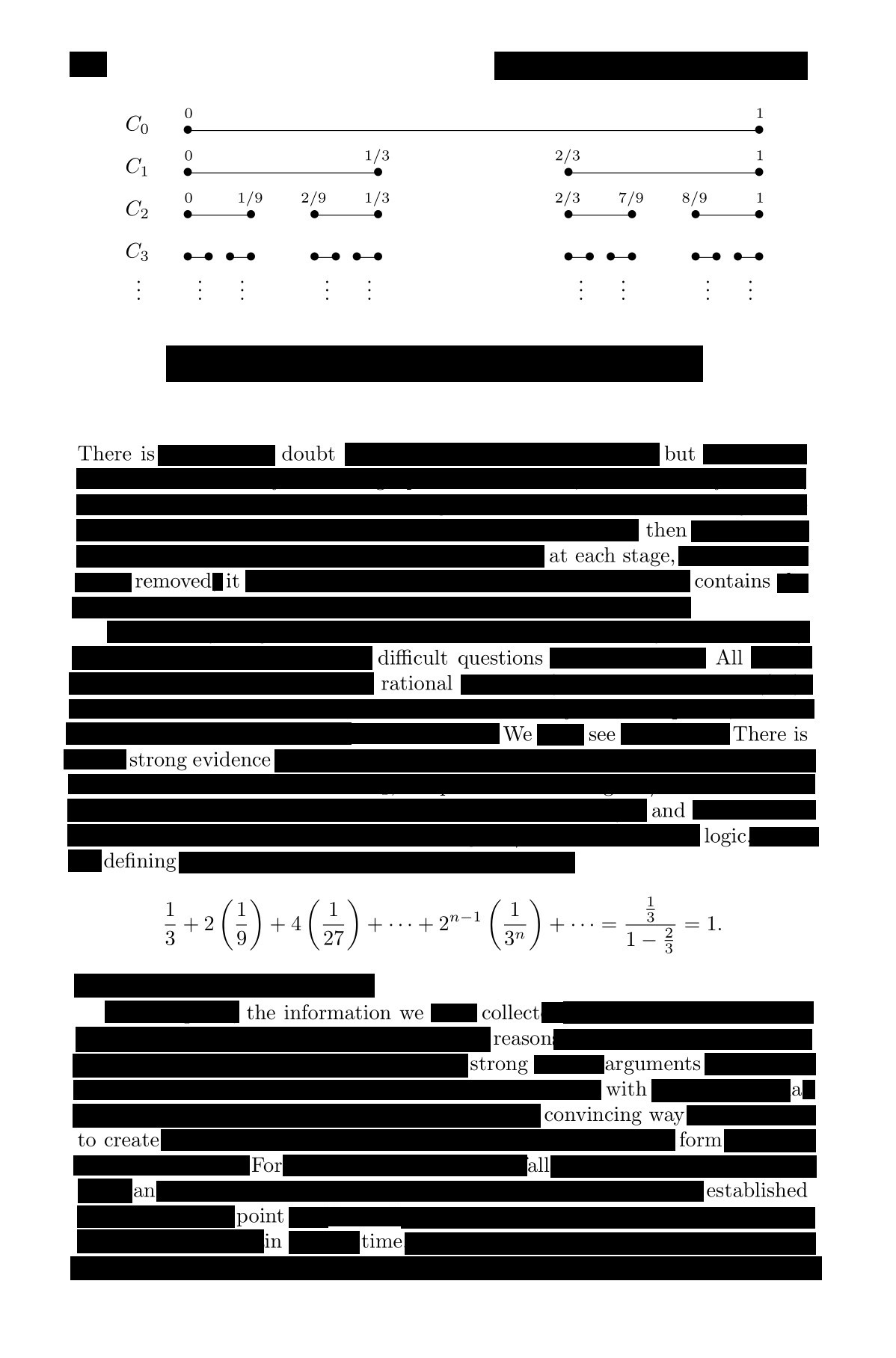"A Real Challenge"

There is doubt
But then at each stage, removed
It contains difficult questions, all rational
We see there is strong evidence and logic
Defining the information we collect
Reason, strong arguments
With a convincing way to create form
For all, an established point in time.

Poem 003: Curvature is Normal (2nd August 2023)
• The Mathematical Topic
• Differential geometry: this topic studies the geometry of curves and surfaces, and is not covered in all undergraduate degree schemes in the UK. Over the years, after various generalisations, it has grown into a large field with applications to many areas of mathematics, as well as to physics, such as the language used to describe Einstein's general theory of relativity. The basic questions asked in an undergraduate course is how do we describe the "curve" (more precisely, curvature) of a curve? What about the curvature of a surface? Perhaps surprisingly, there is more than one way to describe how a surface is curved.

• The Book
• Elementary Differential Geometry
Barrett O'Neill (2006)

(The poem uses page 209 from the revised second edition)

• The Poem: "Curvature is Normal"
•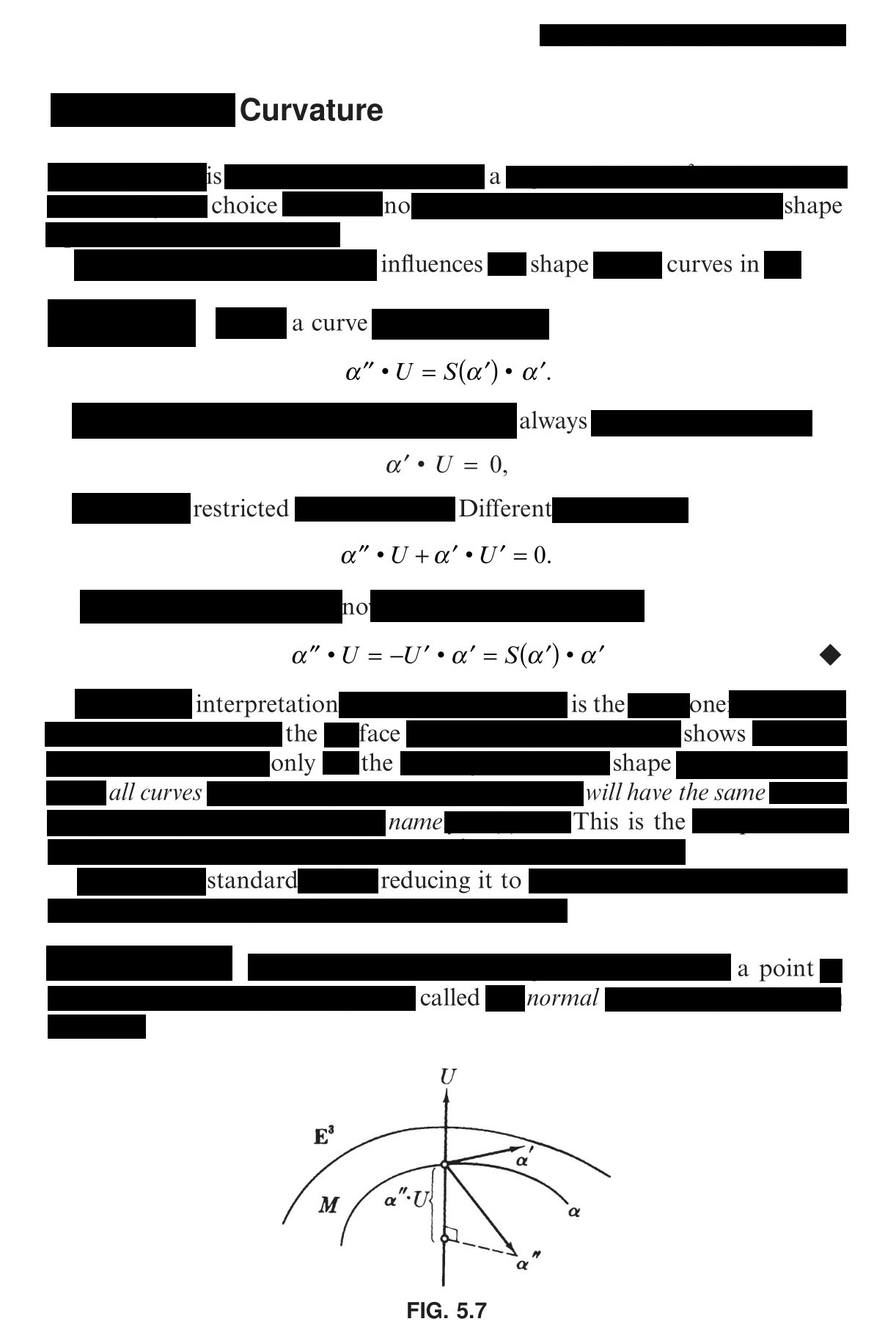"Curvature is Normal"

Curvature is a choice, no?
Shape influences shape
Curves in a curve
Always restricted, different
No interpretation is the one
The face shows only the shape
All curves will have the same name
This is the standard
Reducing it to a point called normal.

Poem 004: Primes (16th August 2023)
• The Mathematical Topic
• Number theory: A fundamental topic in mathematics, number theory is the study of whole numbers and the relationships between them. They are familiar objects and questions can often be easy to state, yet often be suprisingly difficult to answer. A basic concept is that of a prime number, studied in detail still in research-level mathematics despite being an idea introduced to school children.

• The Book
• Discrete Mathematics with Graph Theory
Edgar Goodaire and Michael Parmenter (2017)
Pearson Prentice-Hall: Upper Saddle River, NJ

(The poem uses page 119 from the third edition)

• The Poem: "Primes"
•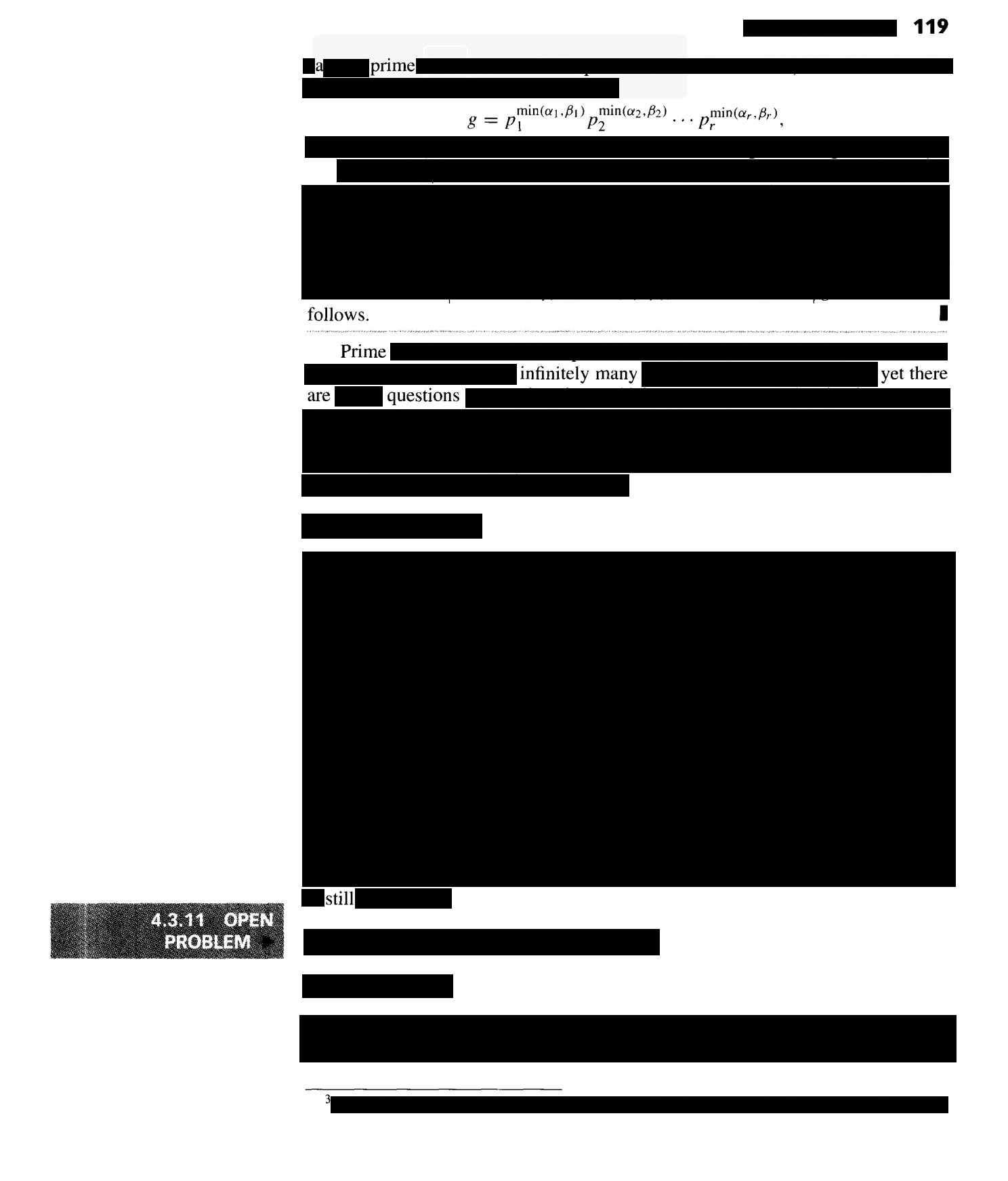"Primes"
A prime follows prime
Infinitely many, yet
There are questions still

Poem 005: Interpolation (30th August 2023)
• The Mathematical Topic
• Numerical analysis: It is not uncommon in mathematics and the physical sciences to encounter a problem that offers no analytical solution. In such cases, one resorts to numerical techniques to understand the situation. The field of numerical analysis is devoted to understanding and applying these methods. A simple example, encountered in most UK undergraduate mathematics degrees, is that of interpolation: estimating unknown data values based upon a given set of known data points.

• The Book
• Numerical Analysis
Rainer Kress (1998)
Springer-Verlag: New York

(The poem uses page 152)

• The Poem: "Interpolation"
•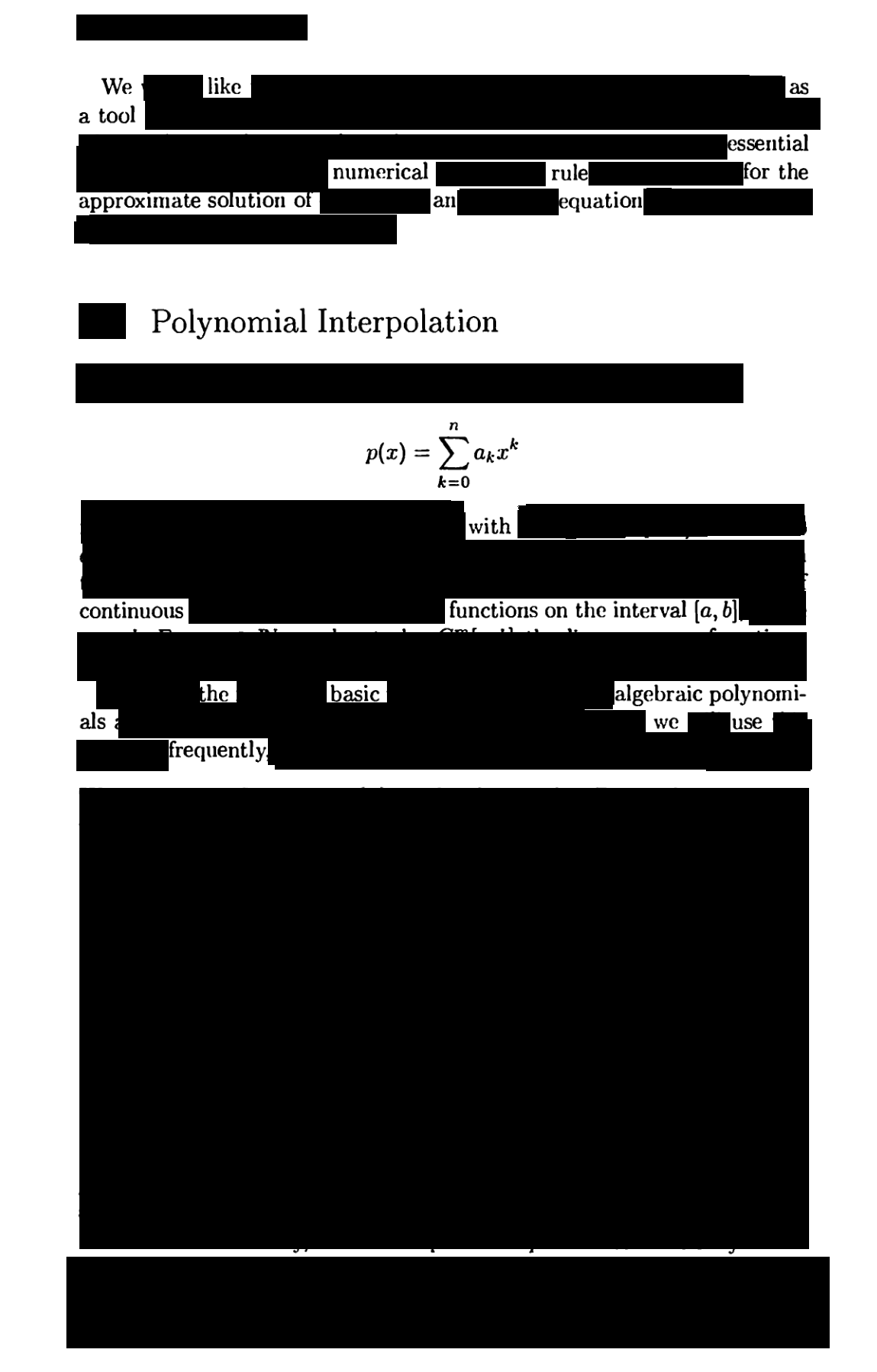"Interpolation"
We like as a tool
An essential numerical rule
For the approximate solution of an equation
Polynomial interpolation
With continuous functions on the interval [a, b]
The basic algebraic polynomials we use frequently

Poem 006: Fourier Knew (13th September 2023)
• The Mathematical Topic
• Fourier analysis: an important branch of mathematics first examined in the 18th century which has wide applicability within physics and engineering. Named after the French mathematician Joseph Fourier, it has been studied and developed for over 200 years and is covered in the majority of UK undergraduate STEM subjects. Historically, it was explored how to represent periodic functions using sums of trigonometric functions and has now been generalised to more abstract situations, leading to the development of new branches of mathematics.

• The Book
• Fourier Analysis
T. W. Korner (2008)
Cambridge University Press: Cambridge

(The poem uses page 3 of the revised edition)

• The Poem: "Fourier Knew"
•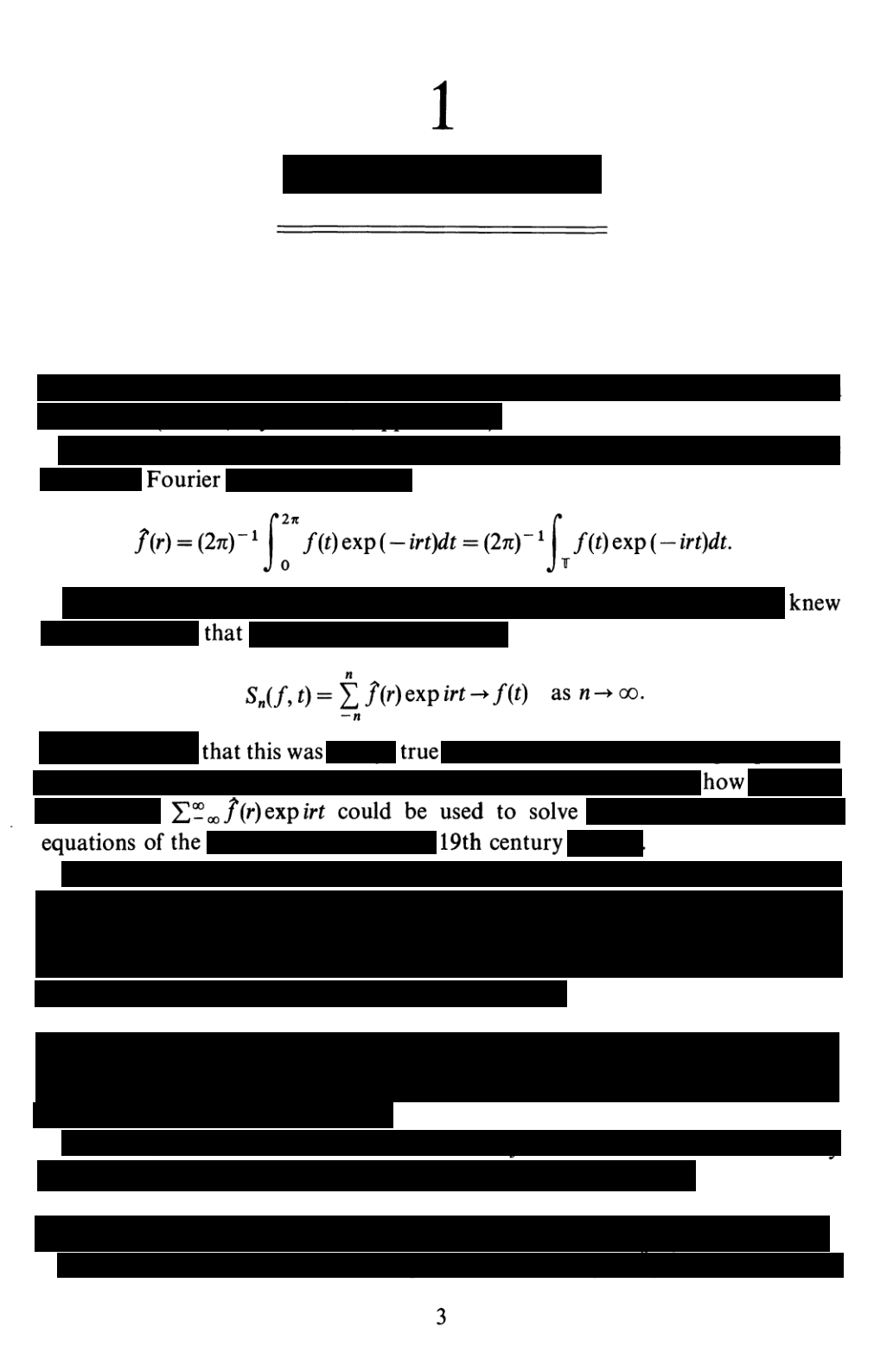"Fourier Knew"
Fourier knew
That this was true
The infinite sum of f(r)exp(irt) [f of r, e to the i r t]
Could be used to solve equations of the 19th century.

Poem 007: Perturbations (27th September 2023)
• The Mathematical Topic
• Perturbation theory: When one is faced with a complex problem in mathematics, one can often build approximate solutions if one can notice "small" terms that relate the complex problem to a much simpler, solvable problem. These solutions, built as a power series in the "small" terms, are called perturbative solutions and this technique - perturbation theory - is used to analyse a wide class of physical problems, from quantum mechanics to fluid dynamics.

• The Book
• Applied Mathematics: A Contemporary Approach
J. D. Logan (1987)
Wiley & Sons: New York

(The poem uses page 34)

• The Poem: "Perturbed"
•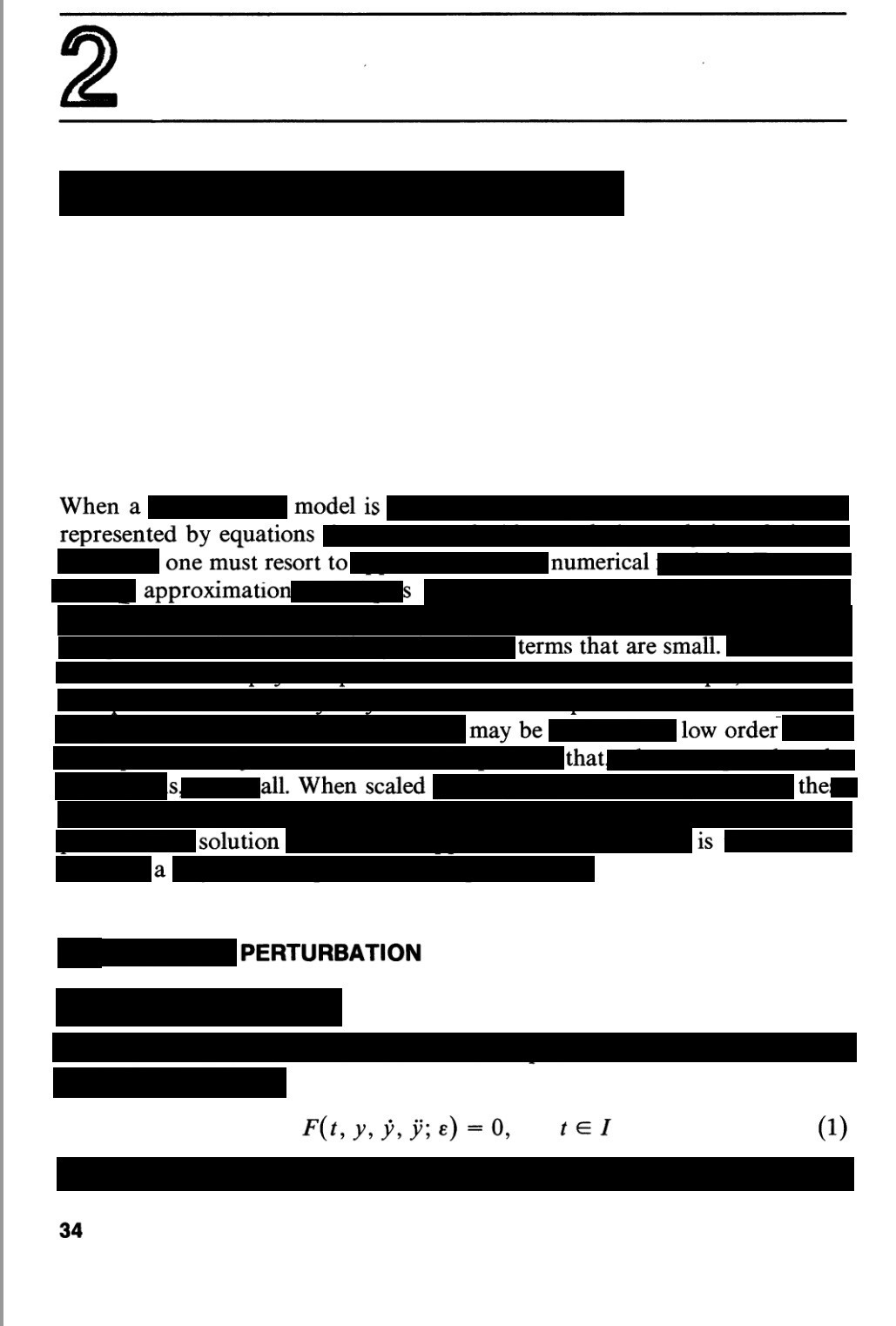"Perturbations"
When a model is represented by equations
One must resort to numerical approximations
Terms that are small
May be low order, that's all
When scaled the solution is a perturbation.

The poem above is a limerick, a traditional, often-humorous English form of verse (although the level of humour here is debatable).

Poem 008: Stokes (11th October 2023)
• The Mathematical Topic
• Stokes' Theorem: Stokes' Theorem is a classical result in vector calculus, an important branch of mathematics covering integration and differentiation of scalar and tensor fields (generally in a Euclidean three-dimensional space). It is often considered a difficult topic and is taught to many undergraduate students in wide range of STEM subjects. Stokes' Theorem can be considered a generalisation of the Fundamental Theorem of Calculus, a result in single variable calculus, to the case of three dimensions. It can, as with the whole topic of vector calculus, be generalised to an arbitrary number of dimensions.

• The Book
• Vector Calculus
S. J. Colley (2011)
Pearson: Boston, MA

(The poem uses page 490 of the 4th edition)

• The Poem: "Stokes"
•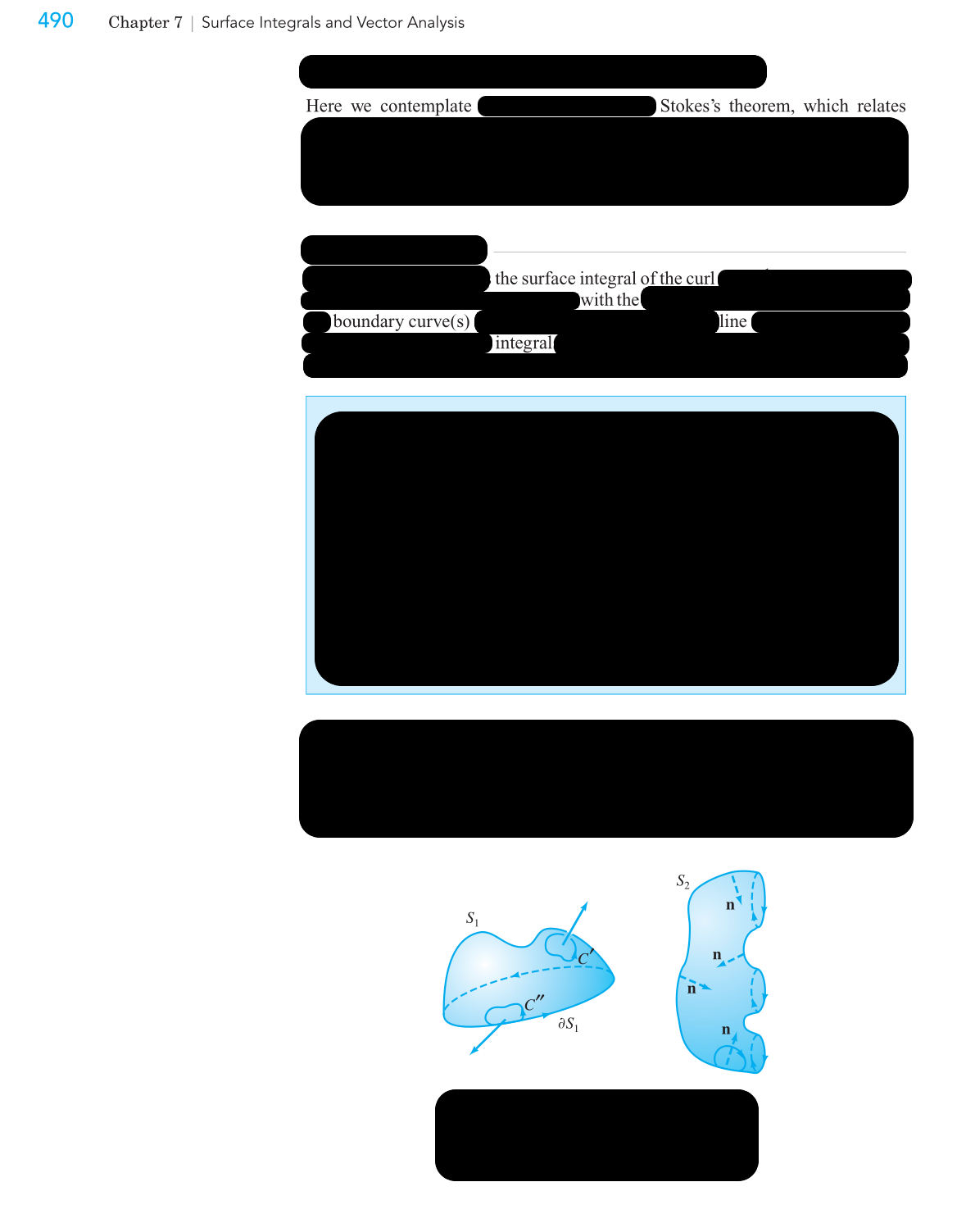"Stokes"
Here we contemplate
Stokes's Theorem, which relates
The surface integral of the curl
With the boundary curve(s) line integral.

Poem 009: (appearing on 25th October 2023)